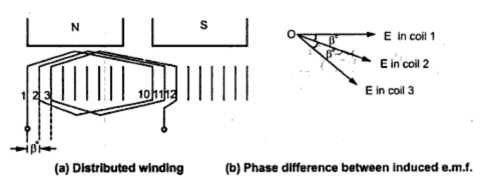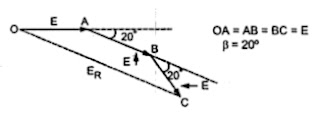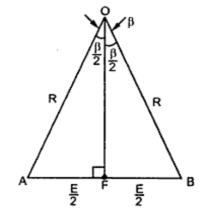### Distribution Factor (Kd)

E.M.F. Equation of an Alternator : Part3
Similar to full pitch coils, concentrated winding is also rare in practice. Attempt is made to use all the slots available under a pole for the winding which makes the nature of the induced e.m.f. more sinusoidal. Such a winding is called distributed winding.
Consider 18 slots, 2 pole alternator. So slots per pole i.e. n = 9.
m = Slots per pole per phase = 3
β = 180o /9 = 20o
Let E = Induced e.m.f. per coil and there are 3 coils per phase.
In concentrated type all the coil sides will be placed in one slot under a pole. So induced e.m.f. in all the coils will achieve maxima and minima at the same time i.e. all of them will be in phase. Hence resultant e.m.f. after connecting coils in series will be algebraic sum of all the e.m.f.s. as all are in phase.
As against this, in distributed type, coil sides will be distributed, one each in the 3 slots per phase available under a pole as shown in the Fig. 1(a).Fig. 1
Thought the magnitude of e.m.f. in each coil will be same as 'E', as each slot contributes phase difference of βo i.e. 20o in this case, there will exist a phase difference of  βo with respect to each other as shown in the Fig. 1(b). Hence resultant e.m.f. will be phasor sum of all of them as shown in the the Fig. 2. So due to distributed winding resultant e.m.f. decreases.Fig. 2 Phasor sum of  e.m.f.s
Note : The factor by which there is a reduction in the e.m.f. due to distribution of coils is called distribution factor denoted as Kd.
Let us see the derivation for its expression.
In general let there be 'n' slots per pole and 'm' slots per pole per phase. So there will be 'm' coils distributed under a pole per phase, connected in series. Let E be the induced e.m.f. per coil. Then all the 'm' e.m.f.s induced in the coils will have successive phase angle difference of β = 180o/n. While finding out the phasor sum of all of them, phasor diagram will approach a shape of a 'm' equal sided polygon circumscribed by a semicircle of radius 'R'.
This is shown in the Fig. 3. AB, BC, CD etc, represents e.m.f. per coil. All the ends joined at 'O' which is center of the circumscribing semicircle of raduis 'R'.Fig. 3  Phasor sum of 'm' e.m.f.s
Angle subtended by each phasor at the origin 'O' is βo. This can be proved as below. All the triangles OAB, OBC .... are similar and isosceles, as AB = BC = CD = ... = E.
∟OAB = ∟OBA = ∟OBC = .... = x
∟AOB = ∟BOC = ... = y say
Now in Δ OAB,        2x + y = 180
while ∟OBA + ∟OBC + β = 180o                             ................. (3)
i.e. 2x + β = 180
Comparing (3) and (4),  y =  β
So      ∟AOB   = ∟BOC  = ∟COD = ... = β
If 'M' is the last point of the last phasor,
∟AOM = m x β = mβ
and AM = ER = Resultant of all the e.m.f.s.
Consider a ΔOAB separately as shown in the Fig. 4. Let OF be the perpendicular drawn on AB bisecting angle at apex 'O' as β/2.Fig. 4
l (AB) = E     ...        l (AF) = E/2
and  l (OA) = R.
...     sin (β/2) = AF/OA = (E/2)/R
...     E = 2R sin (β/2)                                                        ........... (5)
Now consider Δ OAM as shown in the Fig. . 3 and OG is the perpendicular drawn from 'O' on its base bisecting ∟OAM.
...     ∟AOG = ∟GOM = (mβ)/2
...     l (AM) = E
...     l (AG) = E/2
= 2 (OA) sin (mβ/2)
Arithmetic sum of e.m.f.s = Arc AB = OA x (mβ)
Note : The angle (mβ/2) in the denominator must be in radians.
Note : The above formula is used to calculate distribution factor when phase spread is and the winding is uniformly distributed.

Related Articles

- E.M.F. Equation of an Alternator
- Pitch Factor or Coil Span Factor (Kc)
- Generalized Expression for E.M.F. Equation ofan Alternator
- Line Value of Induced E.M.F

1.kd = {sinmb/2}/msinb/2

1.I have tried both (yours and the one above) for an 8 pole, 9-slot machine and got a value greater than 1. What do you think?

2.Galat h upar wala formula
Rohit k name wala shi h forrmula

2.This comment has been removed by the author.

3.I have searched for a plausible explanation to this concept and I found it here. Thanks!!
Please keep up the good work!
Regards!